SAMPLE PROBLEMS
PROBLEMS HOME

LINEAR ALGEBRA
VECTORS
SYSTEM OF LINEAR EQUATIONS
MATRICES
EIGENVALUES & EIGENVECTORS
ORTHOGONALITY
VECTOR SPACE
DISTANCE & APPROXIMATION

MAIN
HOME
TESTS
TUTORIALS
SAMPLE PROBLEMS
COMMON MISTAKES
STUDY TIPS
GLOSSARY
APPLICATIONS
MATH HUMOUR

### VECTOR SPACE PROBLEMS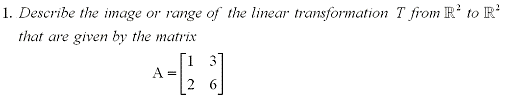Solution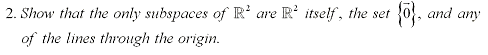Solution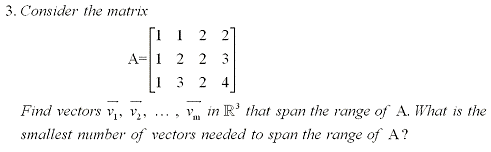Solution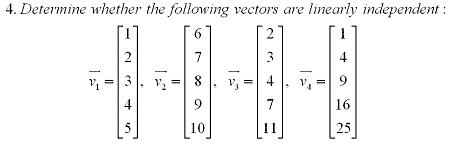Solution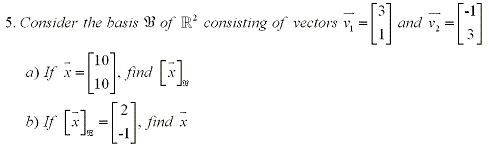Solution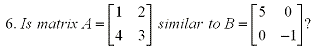Solution Here are some more challenging problems without solutions: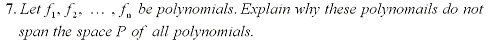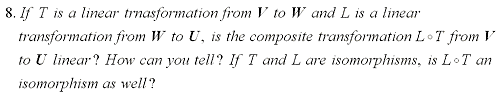If you are having any trouble with these problems, it is recommended that you review the vector space tutorial at the link below. Although these problems are a little more challenging, they can still be solved using the same basic concepts covered in the tutorial and examples. To test your knowledge of these application problems, try taking the general vector space test on the iLrn website or the advanced vector space test at the link below. Vector Space Tutorial General Vector Space Test on iLrn Advanced Vector Space Test

FACULTY HOMEPAGES
Alex Karassev